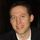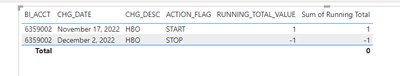cancel
Showing results for
Did you mean:Frequent Visitor

## Filter looking at 2 measures not working.

I can't provide the actual data as some of it is sensitive.

My goal is to look at customers that have positive values for two measured conditions.

Both Cummulative HBO and Total Streaming check to see if an account is active for each service.

I'm trying to write a measure that looks at both and brings back the total count of customers who have both active.

However I just get blanks and I'm not sure why. I've provided a snip-it to illustrate what I'm talking about.

I'd hope that all of those accounts would be flagged.

``````Cummulative HBO =
CALCULATE(
[Sum of Running Total],
'Test Charges Date Table'[Date]<=MAX('Test Charges Date Table'[Date])
, 'TEST CHARGES'[CHG_DESC] in {"HBO"}
)

Cummulative Total Stream =
CALCULATE(
[Sum of Running Total],
'Test Charges Date Table'[Date]<=MAX('Test Charges Date Table'[Date])
, 'TEST CHARGES'[CHG_DESC] in {"Streaming Local Plus", "Streaming Complete"}
)

TEST Combined =
CALCULATE(
DISTINCTCOUNT('TEST CHARGES'[BI_ACCT]),
FILTER('TEST CHARGES',  [Cummulative HBO] = 1 && [Cummulative Total Stream] = 1
)
)``````Any help is appreciated. Thanks!

1 ACCEPTED SOLUTIONSuper User

Thanks for the pbix. Try this measure:

``````Distinct Count Combined =
VAR vRunningTotal =
CALCULATETABLE (
VALUES ( 'TEST CHARGES'[BI_ACCT] ),
"@CumulativeHBO", [Cummulative HBO],
"@CumulativeTotalStream", [Cummulative Total Stream]
),
'Test Charges Date Table'[Date] <= MAX ( 'Test Charges Date Table'[Date] )
)
VAR vActiveCustomers =
SELECTCOLUMNS (
FILTER ( vRunningTotal, [@CumulativeHBO] = 1 && [@CumulativeTotalStream] = 1 ),
"BI_ACCT", 'TEST CHARGES'[BI_ACCT]
)
VAR vResult =
CALCULATE ( DISTINCTCOUNT ( 'TEST CHARGES'[BI_ACCT] ), vActiveCustomers )
RETURN
vResult``````

Proud to be a Super User!

8 REPLIES 8Super User

Try this measure:

``````Distinct Count Combined =
VAR vHBO =
CALCULATETABLE (
VALUES ( 'TEST CHARGES'[BI_ACCT] ),
'Test Charges Date Table'[Date] <= MAX ( 'Test Charges Date Table'[Date] ),
'TEST CHARGES'[CHG_DESC] = "HBO"
)
VAR vStream =
CALCULATETABLE (
VALUES ( 'TEST CHARGES'[BI_ACCT] ),
'Test Charges Date Table'[Date] <= MAX ( 'Test Charges Date Table'[Date] ),
'TEST CHARGES'[CHG_DESC] IN { "Streaming Local Plus", "Streaming Complete" }
)
VAR vBoth =
INTERSECT ( vHBO, vStream )
VAR vResult =
CALCULATE ( DISTINCTCOUNT ( 'TEST CHARGES'[BI_ACCT] ), vBoth )
RETURN
vResult``````

Proud to be a Super User!Frequent Visitor

@DataInsights
Thank you for the quick reply. This is very close but currently brings in any customer whos ever had both services. In my original code the "Sum of Running Total" checks to see if a particular service is active or not.
It's just adding up 1s and -1s where 1 equals active and 0 equals inactive. I filtered each measure to only look at specific charge codes so that it would see if those specific services were active. If no service is designated it just checks if the account itself is active.

``````Sum of Running Total =
SUM('TEST CHARGES'[RUNNING_TOTAL_VALUE])``````

Can I add that calculation to the calculatate table variables?Super User

Try this:

``````Distinct Count Combined =
VAR vHBO =
CALCULATETABLE (
VALUES ( 'TEST CHARGES'[BI_ACCT] ),
'Test Charges Date Table'[Date] <= MAX ( 'Test Charges Date Table'[Date] ),
'TEST CHARGES'[CHG_DESC] = "HBO",
'TEST CHARGES'[RUNNING_TOTAL_VALUE] = 1
)
VAR vStream =
CALCULATETABLE (
VALUES ( 'TEST CHARGES'[BI_ACCT] ),
'Test Charges Date Table'[Date] <= MAX ( 'Test Charges Date Table'[Date] ),
'TEST CHARGES'[CHG_DESC] IN { "Streaming Local Plus", "Streaming Complete" },
'TEST CHARGES'[RUNNING_TOTAL_VALUE] = 1
)
VAR vBoth =
INTERSECT ( vHBO, vStream )
VAR vResult =
CALCULATE ( DISTINCTCOUNT ( 'TEST CHARGES'[BI_ACCT] ), vBoth )
RETURN
vResult``````

Proud to be a Super User!Frequent VisitorI'm hoping this picture helps illustrate the running total value. If we look at
"Running Total Value" = 1 then we still have every account that EVER had service since they all "START" with a 1. In the example we see on Dec 2nd they stopped service and the "Sum of Running Total" is now 0. They can start and stop service as many times as they'd like so we have to always look at the sum of the running totals.

When I try adding the measure to the filter condition I get the placeholder error code.

``````VAR vHBO =
CALCULATETABLE (
VALUES ( 'TEST CHARGES'[BI_ACCT] ),
'Test Charges Date Table'[Date] <= MAX ( 'Test Charges Date Table'[Date] ),
[Sum of Running Total] = 1,
'TEST CHARGES'[CHG_DESC] = "HBO"
)``````If we're able to get it to accept the measure I beleive we'd have the finished product.Super User

Try this. Would you be able to provide sample data (paste as table), and a screenshot of the expected result? This will facilitate testing.

``````Distinct Count Combined =
VAR vRunningTotal =
CALCULATETABLE (
SUMMARIZE ( 'TEST CHARGES', 'TEST CHARGES'[BI_ACCT], 'TEST CHARGES'[CHG_DESC] ),
"@RunningTotal", [Sum of Running Total]
),
'Test Charges Date Table'[Date] <= MAX ( 'Test Charges Date Table'[Date] )
)
VAR vActiveCustomers =
SELECTCOLUMNS (
FILTER ( vRunningTotal, [@RunningTotal] = 0 ),
"BI_ACCT", 'TEST CHARGES'[BI_ACCT]
)
VAR vHBO =
CALCULATETABLE (
VALUES ( 'TEST CHARGES'[BI_ACCT] ),
'Test Charges Date Table'[Date] <= MAX ( 'Test Charges Date Table'[Date] ),
'TEST CHARGES'[CHG_DESC] = "HBO",
vActiveCustomers
)
VAR vStream =
CALCULATETABLE (
VALUES ( 'TEST CHARGES'[BI_ACCT] ),
'Test Charges Date Table'[Date] <= MAX ( 'Test Charges Date Table'[Date] ),
'TEST CHARGES'[CHG_DESC] IN { "Streaming Local Plus", "Streaming Complete" },
vActiveCustomers
)
VAR vBoth =
INTERSECT ( vHBO, vStream )
VAR vResult =
CALCULATE ( DISTINCTCOUNT ( 'TEST CHARGES'[BI_ACCT] ), vBoth )
RETURN
vResult``````

Proud to be a Super User!Super User

Correction (wrong value for RunningTotal):

``````Distinct Count Combined =
VAR vRunningTotal =
CALCULATETABLE (
SUMMARIZE ( 'TEST CHARGES', 'TEST CHARGES'[BI_ACCT], 'TEST CHARGES'[CHG_DESC] ),
"@RunningTotal", [Sum of Running Total]
),
'Test Charges Date Table'[Date] <= MAX ( 'Test Charges Date Table'[Date] )
)
VAR vActiveCustomers =
SELECTCOLUMNS (
FILTER ( vRunningTotal, [@RunningTotal] = 1 ),
"BI_ACCT", 'TEST CHARGES'[BI_ACCT]
)
VAR vHBO =
CALCULATETABLE (
VALUES ( 'TEST CHARGES'[BI_ACCT] ),
'Test Charges Date Table'[Date] <= MAX ( 'Test Charges Date Table'[Date] ),
'TEST CHARGES'[CHG_DESC] = "HBO",
vActiveCustomers
)
VAR vStream =
CALCULATETABLE (
VALUES ( 'TEST CHARGES'[BI_ACCT] ),
'Test Charges Date Table'[Date] <= MAX ( 'Test Charges Date Table'[Date] ),
'TEST CHARGES'[CHG_DESC] IN { "Streaming Local Plus", "Streaming Complete" },
vActiveCustomers
)
VAR vBoth =
INTERSECT ( vHBO, vStream )
VAR vResult =
CALCULATE ( DISTINCTCOUNT ( 'TEST CHARGES'[BI_ACCT] ), vBoth )
RETURN
vResult``````

Proud to be a Super User!Frequent Visitor

Here is a link to a sample file I prepared. Within I have a few example as well as (hopefully) details as to what the final product needs to be.

If you need anything else or the link doesn't work please let me know. Thank you very much for your assistance.Super User

Thanks for the pbix. Try this measure:

``````Distinct Count Combined =
VAR vRunningTotal =
CALCULATETABLE (
VALUES ( 'TEST CHARGES'[BI_ACCT] ),
"@CumulativeHBO", [Cummulative HBO],
"@CumulativeTotalStream", [Cummulative Total Stream]
),
'Test Charges Date Table'[Date] <= MAX ( 'Test Charges Date Table'[Date] )
)
VAR vActiveCustomers =
SELECTCOLUMNS (
FILTER ( vRunningTotal, [@CumulativeHBO] = 1 && [@CumulativeTotalStream] = 1 ),
"BI_ACCT", 'TEST CHARGES'[BI_ACCT]
)
VAR vResult =
CALCULATE ( DISTINCTCOUNT ( 'TEST CHARGES'[BI_ACCT] ), vActiveCustomers )
RETURN
vResult``````

Proud to be a Super User!